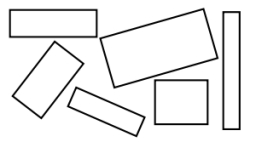# Rectangular 26641

The area of the work surface of the rectangular table is 70 dm2, and its perimeter is 34 dm. Determine (in dm) the length of the shorter side of this table.

a =  7 dm

### Step-by-step explanation:Did you find an error or inaccuracy? Feel free to write us. Thank you!

Tips for related online calculators
Are you looking for help with calculating roots of a quadratic equation?
Do you have a linear equation or system of equations and looking for its solution? Or do you have a quadratic equation?

#### Grade of the word problem:

We encourage you to watch this tutorial video on this math problem: